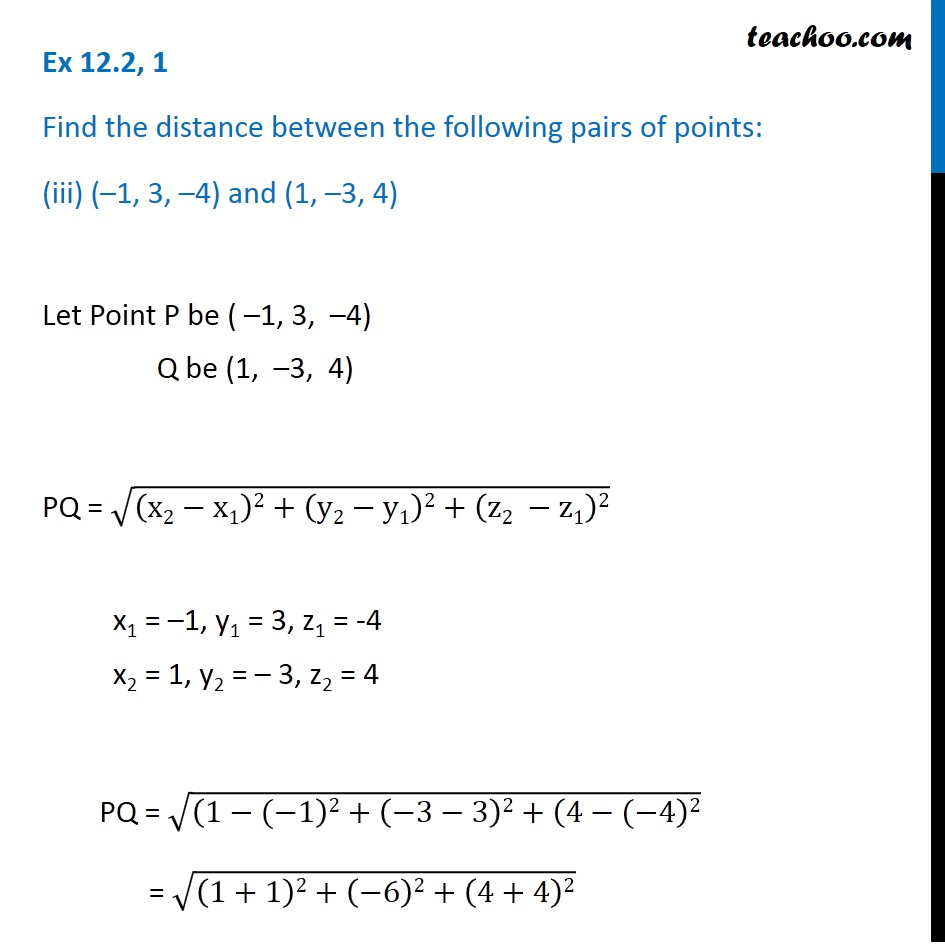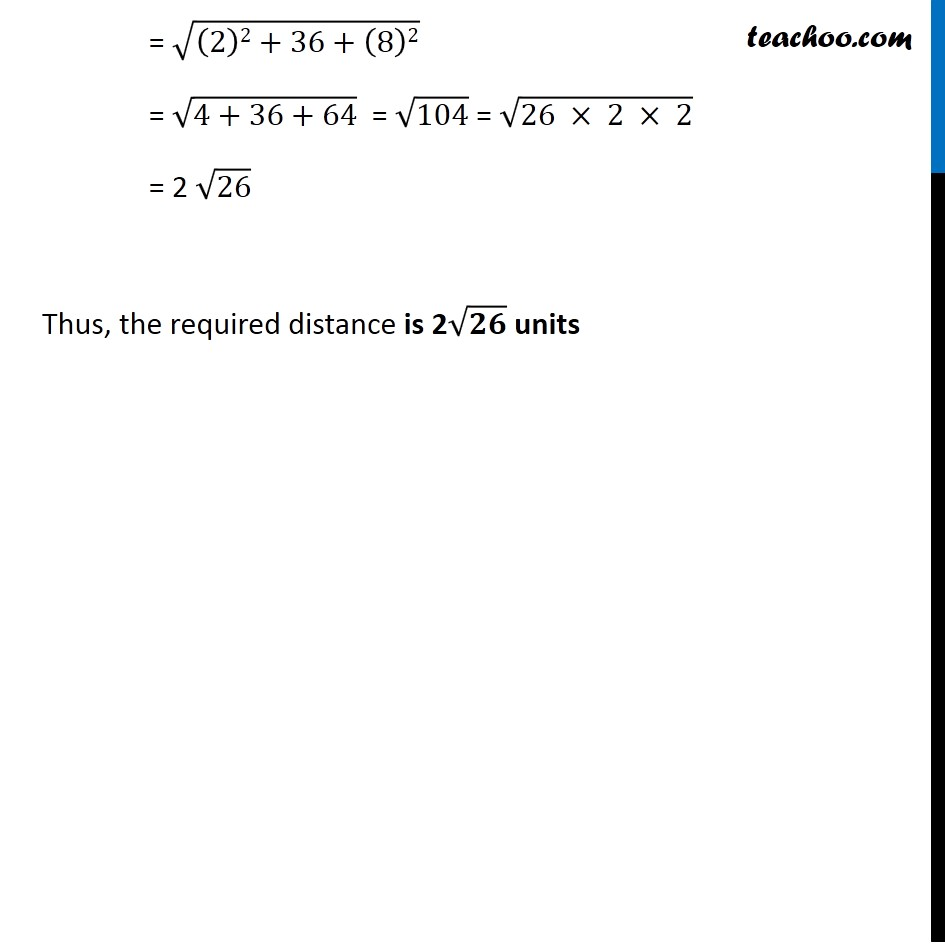Ex 11.2

Chapter 11 Class 11 - Intro to Three Dimensional Geometry
Serial order wiseLearn in your speed, with individual attention - Teachoo Maths 1-on-1 Class

### Transcript

Ex 11.2, 1 Find the distance between the following pairs of points: (iii) (–1, 3, –4) and (1, –3, 4) Let Point P be ( –1, 3, –4) Q be (1, –3, 4) PQ = √((x2−x1)2+(y2−y1)2+(z2 −z1)2) x1 = –1, y1 = 3, z1 = -4 x2 = 1, y2 = – 3, z2 = 4 PQ = √((1−(−1)2+(−3−3)2+(4−(−4)2) = √((1+1)2+(−6)2+(4+4)2) = √((2)2+36+(8)2) = √(4+36+64) = √104 = √(26 × 2\ × 2) = 2 √26 Thus, the required distance is 2√𝟐𝟔 units